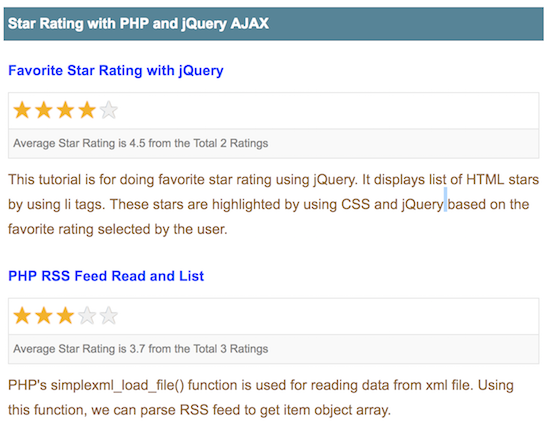# Star Rating with PHP and jQuery AJAX

Star rating is used to rank pages, articles or posts published to the end user. This will help the user to identify and shortlist quality contents that are highly ranked. In this article, we are going to see how to create a PHP star rating system using jQuery AJAX. This article is an improved version of the existing linked code. In this example, I have calculated the average star rating from the total ratings added for each tutorial.This star rating example shows clickable five stars for each tutorial to add rating. The tutorials and their rating are stored in the database. I have stored the rating based on the member session. I hardcoded the member session id at the first line of the program. You can integrate your authentication module to replace this by the logged in user session id.

View Demo

## List Tutorial with Star Rating

This code is used to retrieve the tutorial data from the database and to list them with the star rating. I combine the tables tutorials and tbl_member_rating using LEFT JOIN to get the star rating along with the tutorial information. For each tutorial, I display the rating added by the user with the graphical representation of highlighting the stars. Also, I calculate the average rating out of 5 by using the overall rating added for the tutorial.

```<?php
\$member_id = 1;
require_once ("Rate.php");
\$rate = new Rate();
\$result = \$rate->getAllPost();

if (! empty(\$result)) {
\$i = 0;
foreach (\$result as \$tutorial) {
\$ratingResult = \$rate->getRatingByTutorialForMember(\$tutorial["id"], \$member_id);
\$ratingVal = "";
if (! empty(\$ratingResult["rating"])) {
\$ratingVal = \$ratingResult["rating"];
}
?>
<tr>
<td valign="top">
<div class="feed_title"><?php echo \$tutorial["title"]; ?></div>
<div id="tutorial-<?php echo \$tutorial["id"]; ?>"
class="star-rating-box">
<input type="hidden" name="rating" id="rating"
value="<?php echo \$ratingVal; ?>" />
<ul
onMouseOut="resetRating(<?php echo \$tutorial["id"]; ?>);">
<?php
for (\$i = 1; \$i <= 5; \$i ++) {
\$selected = "";
if (! empty(\$ratingResult["rating"]) && \$i <= \$ratingResult["rating"]) {
\$selected = "selected";
}
?>
<li class='<?php echo \$selected; ?>'
onmouseover="highlightStar(this,<?php echo \$tutorial["id"]; ?>);"
onmouseout="removeHighlight(<?php echo \$tutorial["id"]; ?>);"
<?php }  ?>
</ul>
<div
id="star-rating-count-<?php echo \$tutorial["id"]; ?>"
class="star-rating-count">
<?php

if (! empty(\$tutorial["rating_total"])) {
\$average = round((\$tutorial["rating_total"] / \$tutorial["rating_count"]), 1);
echo "Average Star Rating is " . \$average . " from the Total " . \$tutorial["rating_count"] . " Ratings";
?>

<?php } else { ?>
No Ratings
<?php  } ?>
</div>

</div>
<div><?php echo \$tutorial["description"]; ?></div>
</td>
</tr>
<?php
}
}
?>
```

## Add Rating via jQuery AJAX

This jQuery code shows the functions used to handle the events triggered during the process of adding the star rating. For example, handling the hover effect to highlight and reset the star rating icons or handling the click event to add rating. The function addRating() is called on selecting the star icon to rate a tutorial. This function will send a request to the PHP code for adding the star rating for a particular tutorial with the reference of the tutorial id.

```<script src="jquery-3.2.1.min.js" type="text/javascript"></script>
<script>
function highlightStar(obj, id) {
removeHighlight(id);
\$('.demo-table #tutorial-' + id + ' li').each(function(index) {
if (index == \$('.demo-table #tutorial-' + id + ' li').index(obj)) {
return false;
}
});
}

function removeHighlight(id) {
\$('.demo-table #tutorial-' + id + ' li').removeClass('selected');
\$('.demo-table #tutorial-' + id + ' li').removeClass('highlight');
}

\$('.demo-table #tutorial-' + id + ' li').each(function(index) {
\$('#tutorial-' + id + ' #rating').val((index + 1));
if (index == \$('.demo-table #tutorial-' + id + ' li').index(obj)) {
return false;
}
});
\$.ajax({
data : 'id=' + id + '&rating='
+ \$('#tutorial-' + id + ' #rating').val(),
type : "POST",
success : function(data) {
\$("#star-rating-count-" + id).html(data);
}
});
}

function resetRating(id) {
if (\$('#tutorial-' + id + ' #rating').val() != 0) {
\$('.demo-table #tutorial-' + id + ' li').each(function(index) {
if ((index + 1) == \$('#tutorial-' + id + ' #rating').val()) {
return false;
}
});
}
}
</script>
```

## PHP Code to Add / Update Star Rating

In PHP code it receives the rating data with the tutorial id. If the tutorial is rated already by the member, then the existing record will be updated with the new rating. Otherwise, a new record will be added to the tbl_member_rating database table to store the user rating. The code for firing the insert or update on the rating database is,

```<?php
\$member_id = 1;
if (! empty(\$_POST["rating"]) && ! empty(\$_POST["id"])) {
require_once ("Rate.php");
\$rate = new Rate();

\$ratingResult = \$rate->getRatingByTutorial(\$_POST["id"], \$member_id);

if (! empty(\$ratingResult)) {
\$rate->updateRating(\$_POST["rating"], \$ratingResult["id"]);
} else {
}

\$postRating = \$rate->getRatingByTutorial(\$_POST["id"]);

if (! empty(\$postRating["rating_total"])) {
\$average = round((\$postRating["rating_total"] / \$postRating["rating_count"]), 1);
echo "Average Star Rating is " . \$average . " from the Total " . \$postRating["rating_count"] . " Ratings";
} else {
echo "No Ratings";
}
}
?>
```

## Star Rating with PHP and jQuery AJAX Output

This screenshot shows the star rating by highlighting the star icons. Also, it shows the average and the total ratings added for each tutorial in the database.